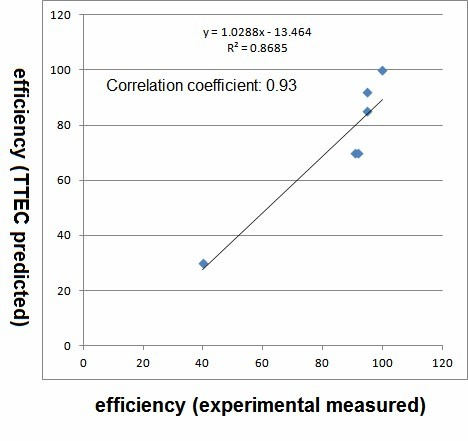Title

# Result

### 1. Fluorescence microscope results

Our plasmid design contains a RFP and GFP. Fluorescence microscope pictures Figure 1 shows that GFP is expressed.(Figure 1 Those bacteria who express GFP is seen under the fluorescence microscope activated by 488nm light.The picture shows the expression of the GFP. )

### 2.Flow cytometry resultsFigure2-aFigure2-b

Flow cytometry results of GFP expression in experiment group Figure2-a and control group Figure2-b.The average GFP fluorescence strength of control group is 432 unit and of experiment group is 251 unit. Therefore, the terminator efficiency is 251/432=0.58

### 3.Agreement with theoretical prediction(Figure 3 The horizontal ordinate represents the value of efficiencies measured by experiment. The vertical ordinate represents the value of efficiencies predicted by TTEC. We can see a good agreement between experimental efficiency and TTEC predicted efficiency.)(Figure 4 Here listed the sequences of terminator whose efficiencies are measured in the lab.)